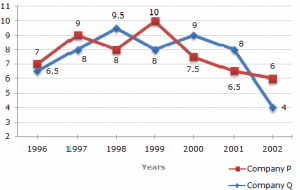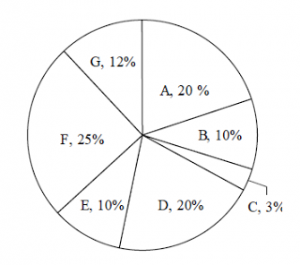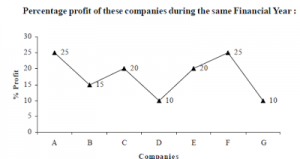## Data Interpretation for SBI PO : Set 04

Directions: 1-5) Two different finance companies declare fixed annual rate of interest on the amounts invest with them by investors. The rate of interest offered by these companies may differ from year to year depending on the variation in the economy of the country and the banks rate of interest. The annual rate of interest offered by the two Companies P and Q over the years is shown by the line graph provided below.

Annual Rate of Interest Offered by Two Finance Companies Over the Years.1) A sum of Rs. 4.75 lakhs was invested in Company Q in 1999 for one year. How much more interest would have been earned if the sum was invested in Company P?

a) Rs 19,000

b) Rs.14, 250

c) Rs.11, 750

d) Rs. 9,500

e) None of these

d) Rs. 9,500
difference = Rs. [(10% of 4.75) – (8% of 4.75)] = Rs. (2% of 4.75) lakhs
= Rs. 0.095 lakhs
= Rs. 9500.

2) If two different amounts in the ratio 8:9 are invested in Companies P and Q respectively in 2002, then the amounts received after one year as interests from Companies P and Q are respectively in the ratio?

a) 2:3

b) 3:4

c) 6:7

d) 4:3

e) None of these

d) 4:3
Let the amounts invested in 2002 in Companies P and Q be Rs. 8x and Rs. 9xrespectively.
Then, interest received after one year from Company P= Rs. (6% of 8x)=Rs.48x/100
and interest received after one year from Company Q= Rs. (4% of 9x)=Rs.36x/100
Required ratio =(48x/100)/(36x/100)=4/3

3) In 2000, a part of Rs. 30 lakhs was invested in Company P and the rest was invested in Company Q for one year. The total interest received was Rs. 2.43 lakhs. What was the amount invested in Company P?

a) 9 lakh

b) 11 lakh

c) Rs. 12 lakh

d) Rs.18 lakh

e) None of these

d) Rs.18 lakh
Let Rs. x lakhs be invested in Company P in 2000, the amount invested in Company Q in
2000 = Rs. (30 – x) lakhs.
Total interest received from the two Companies after 1 year
= Rs. [7.5% of x + 9% of (30 – x)] lakhs
= Rs. 2.7 -1.5x/100 lakhs.
x = 18.

4) An investor invested a sum of Rs. 12 lakhs in Company P in 1998. The total amount received after one year was re-invested in the same Company for one more year. The total appreciation received by the investor on his investment was?

a) 2, 96,200

b) 2, 42,200

c) 2, 25,600

d) 2, 16,000

e) None of these

c) 2, 25,600
Amount received from Company P after one year (i.e., in 1999) on investing Rs. 12 lakhs in it
= Rs. [12 + (8% of 12)] lakhs
= Rs. 12.96 lakhs.
Appreciation received on investment during the period of two years
= Rs. (14.256 – 12) lakhs
= Rs. 2.256 lakhs = Rs. 2, 25,600.

5) An investor invested Rs. 5 lakhs in Company Q in 1996. After one year, the entire amount along with the interest was transferred as investment to Company P in 1997 for one year. What amount will be received from Company P, by the investor?

a) 5, 94,550

b) 5, 80,425

c) 5, 77,800

d) 5, 77,500

e) None of these

b) 5, 80,425
Amount received from Company Q after one year on investment of Rs. 5 lakhs in the year1996
= Rs. [5 + (6.5% of 5)] lakhs
= Rs. 5.325 lakhs.
Amount received from Company P after one year on investment of Rs. 5.325 lakhs in theyear 1997
= Rs. [5.325 + (9% of 5.325)] lakhs
= Rs. 5.80425 lakhs
= Rs. 5, 80, 425.

Directions.6-10) Study the following information carefully and answer the question given below it :

Percentage proportion of income of seven companies in a state during financial year –2013-20146) If the income of company D and B together is Rs. 270 crore then find the profit of company B in that year ?

a) Rs. 14.25 crore

b) Rs. 15.5 crore

c) Rs. 11.75 crore

d) Rs. 12.5 crore

e) None of these

c) Rs. 11.75 crore
(20% + 10%) = 270
10% = 90
Ib = 90

Eb =  (90×100)/115  = 78.26
Pb = 11.739

7) If the expenditure of company D is 750 crore, what was the total income of all the given companies together ?

a) Rs. 4135 crore

b) Rs. 4115 crore

c) Rs. 4125 crore

d) Rs. 4025 crore

e) None of these

c) Rs. 4125 crore

20% ×  100/110  × total = 750

Total = 4125

8) If in the given financial year income of company A was 440 crore. What was the approximately expenditure of company C in that year ?

a) Rs. 45 crore

b) Rs. 55 crore

c) Rs. 46 crore

d) Rs. 35 crore

e) None of these

b) Rs. 55 crore

20% = 440

% =  440/20  = 22

3% = 66 crore

Exp= 66 ×  100/120  = 55 crore

9) What is the ratio of the expenditure of company D to company C ?

a) 80 : 11

b) 11 : 80

c) 12 : 70

d) 70 : 12

e) None of these

a) 80 : 11

10) If the difference between the income of company F and company A is Rs. 750 crore, what is the expenditure of both the companies together ?

a) Rs. 6400 crore

b) Rs. 7400 crore

c) Rs. 8400 crore

d) Rs. 5400 crore

e) None of these